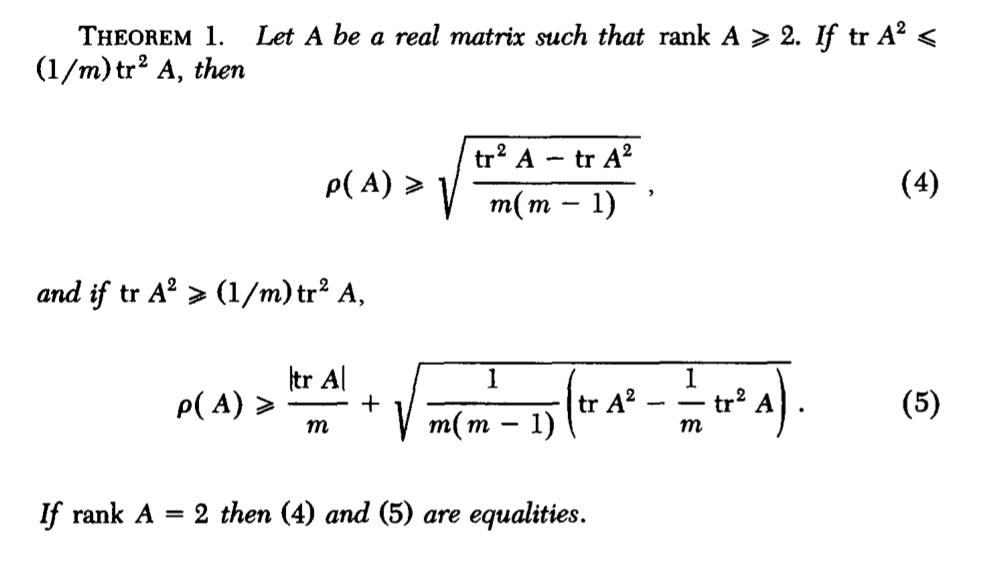# Survey papers on spectral radius [closed]

Let $$M$$ be a $$n\times n$$ matrix.

Are there any survey papers which give lower and upper bounds on its spectral radius?

What are the ways to find some lower bounds and upper bounds on $$\rho(M)$$ except using the fact that $$\rho(A)\ge \frac{x^TMx}{x^Tx}$$?

If someone could provide any way to find bounds or share any recent survey paper, I would be really grateful

• Typo? Is $A = M$? – Geoff Robinson Jan 8 at 10:35
• @GeoffRobinson; yes – Math_Freak Jan 8 at 10:45
• The "fact" that $\rho(M)\ge \frac{x^TMx}{x^Tx}$ is not correct for general non-normal matrices; consider for instance $M = \begin{pmatrix} 0& 1 \\ 0 & 0 \end{pmatrix}$. – Jochen Glueck Jan 8 at 11:06
• I think your question is based on a false premise. There is not going to be a survey paper on something so general, especially since you seem to have overlooked nilpotent matrices (as @JochenGlueck has pointed out). If your underlying question is about getting lower bounds on tthe spectral radius, then you should edit your question to remove the stuff about survey papers – Yemon Choi Jan 8 at 14:51
• @YemonChoi: Small remark: The problem is not so much about nilpotent matrices only, but rather about the fact that the supremum of $\frac{\overline{x}^T M x}{\overline{x}^Tx}$ over all non-zero complex $x$ is the numerical radius rather than the spectral radius - and for non-normal matrices the numerical range can be considerably larger than the convex hull of the spectrum, so the numerical radius can be much larger than the spectral radius. (Of course, this comment does not contradict your remark that the question is very broad.) – Jochen Glueck Jan 8 at 16:13

If the matrix is not positive definite, this lower bound might be useful [source]: